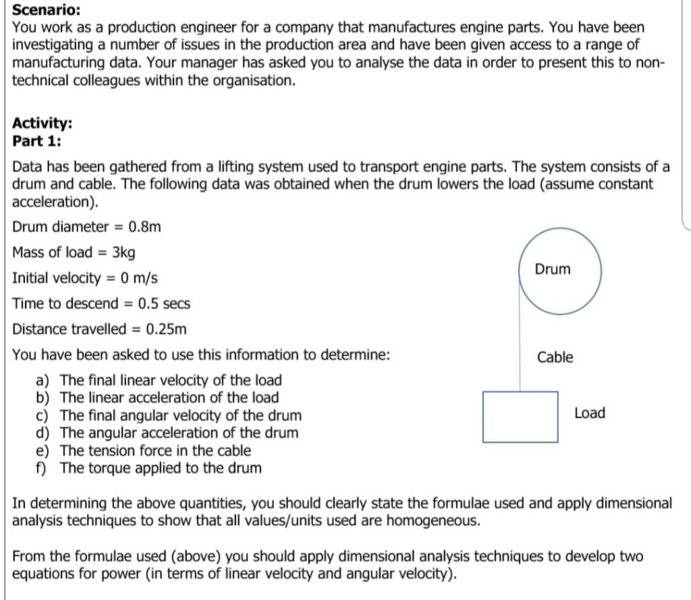# Drum and cable problem

Moved from a technical forum, no template.
Hi, I'm studying a hnc in mechanical engineering.
One of the maths problems I have been given involves a drum and cable. It states the diameter(0.8m), mass (3kg), initial velocity (0m/s), time to descend (0.5secs) and distance travelled (0.25m)

I need to find:
A)final linear velocity of the load
B) linear acceleration of the load
C) final angular velocity of the drum
D) angular acceleration of the drum
E) tension force of the cable
F) torque applied to the drum.

So far, I've got linear and normal velocity and acceleration wrapped around my head. I did a problem similar to this last year with a mass on either side of the pulley, but I'm struggling on how to change that to only having one mass to calculate.

Am I right in thinking V= u+at
V= 0+(2×0.5)
V=1m/s

A=2s/t(squared)- 2u/t
A= 2m/s (squared)?

Like I said, I'm just a little confused as to whether I'm using the correct formulas to find the basics before I start doing the rest of the question.

Thanks in advance for any guidance! :)#### Attachments

haruspex
Homework Helper
Gold Member
Am I right in thinking V= u+at
V= 0+(2×0.5)
V=1m/s

A=2s/t(squared)- 2u/t
A= 2m/s (squared)?
Looks fine.

CWatters
Homework Helper
Gold Member
I did a problem similar to this last year with a mass on either side of the pulley, but I'm struggling on how to change that to only having one mass to calculate.

Hint. This problem is actually easier than last years due to the amount of information provided about how it moves.

Hi, I'm studying a hnc in mechanical engineering.
One of the maths problems I have been given involves a drum and cable. It states the diameter(0.8m), mass (3kg), initial velocity (0m/s), time to descend (0.5secs) and distance travelled (0.25m)

I need to find:
A)final linear velocity of the load
B) linear acceleration of the load
C) final angular velocity of the drum
D) angular acceleration of the drum
E) tension force of the cable
F) torque applied to the drum.

So far, I've got linear and normal velocity and acceleration wrapped around my head. I did a problem similar to this last year with a mass on either side of the pulley, but I'm struggling on how to change that to only having one mass to calculate.

Am I right in thinking V= u+at
V= 0+(2×0.5)
V=1m/s

A=2s/t(squared)- 2u/t
A= 2m/s (squared)?

Like I said, I'm just a little confused as to whether I'm using the correct formulas to find the basics before I start doing the rest of the question.

Thanks in advance for any guidance! :) View attachment 232243
Hi ebony did you manage to complete this..I have the same question and don't know where to begin the response you are asking about I assume is the answer to part a and b...

Hi,

I am just wondering if somebody can help me with this question also. I have been given it, but the time to descend is unknown. I have the other values, with the distance travelled, mass, initial velocity of 0m/s, and the drum diameter. It says to assume constant acceleration, but I am unsure where to start.

PeroK
Homework Helper
Gold Member
2021 Award
Hi,

I am just wondering if somebody can help me with this question also. I have been given it, but the time to descend is unknown. I have the other values, with the distance travelled, mass, initial velocity of 0m/s, and the drum diameter. It says to assume constant acceleration, but I am unsure where to start.It might be best to start a new thread. In any case, you need to show us your own best attempt. See the homework guidelines:

Hi,

I am just wondering if somebody can help me with this question also. I have been given it, but the time to descend is unknown. I have the other values, with the distance travelled, mass, initial velocity of 0m/s, and the drum diameter. It says to assume constant acceleration, but I am unsure where to start.